# Properties of Circles Chapter Exam

Exam Instructions:

Choose your answers to the questions and click 'Next' to see the next set of questions. You can skip questions if you would like and come back to them later with the yellow "Go To First Skipped Question" button. When you have completed the practice exam, a green submit button will appear. Click it to see your results. Good luck!

### Page 1

#### Question 1 1. If AB and CB are tangent segments, what is the length of CB?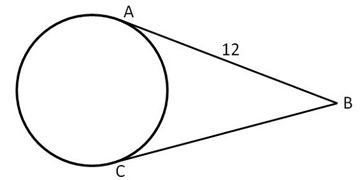#### Question 3 3. If angle AOB is 40 degrees, what is the measure of angle ACB?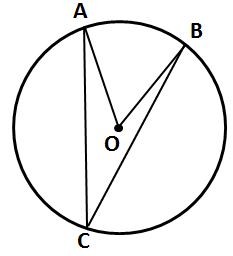### Page 2

#### Question 6 6. If angle ACB is 47 degrees, what is the measure of angle AOB?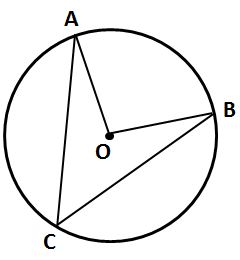#### Question 7 7. Choose the equation for the following circle: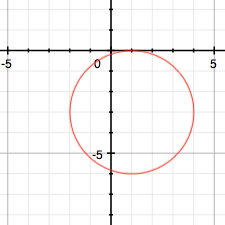#### Question 8 8. In the pictured circle, the measure of angle ACB is 22 degrees and the measure of angle BXD is 120 degrees. What is the measure of angle CAD?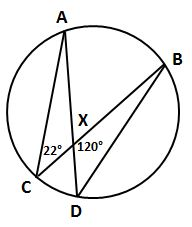#### Question 10 10. What is the area of the shaded area, rounded to the nearest whole number?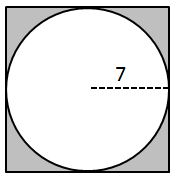### Page 3

#### Question 12 12. Find the center and radius of the following equation: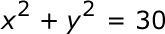#### Question 13 13. Find the center and radius of the following equation: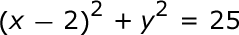#### Question 14 14. If the area of the square is 64, what is the area of the circle, rounded to the nearest whole number?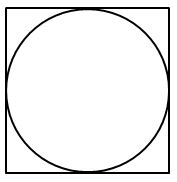#### Question 15 15. Find the center and radius of the following equation: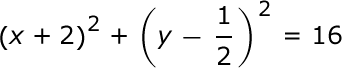### Page 4

#### Question 17 17. What is the area of the shaded area, rounded to the nearest whole number?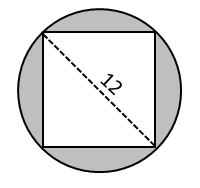#### Question 20 20. Which line is a tangent to the pictured circle?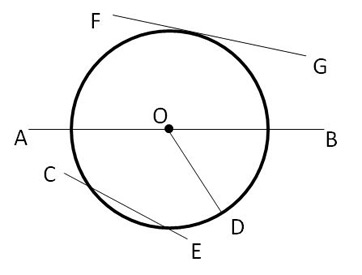### Page 5

#### Question 22 22. If angle ACB is 34 degrees, what is the measure of angle ADB?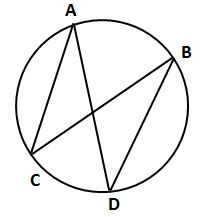### Page 6

#### Question 28 28. If angle OAB is 45 degrees, what is the measure of angle AOB?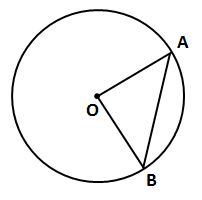#### Properties of Circles Chapter Exam Instructions

Choose your answers to the questions and click 'Next' to see the next set of questions. You can skip questions if you would like and come back to them later with the yellow "Go To First Skipped Question" button. When you have completed the practice exam, a green submit button will appear. Click it to see your results. Good luck!

Support